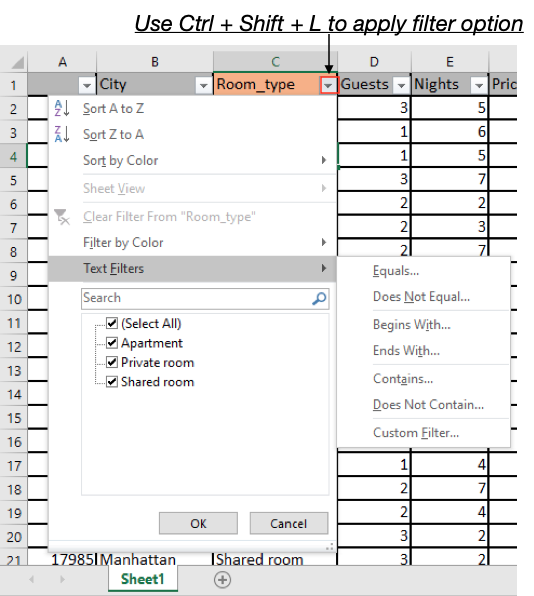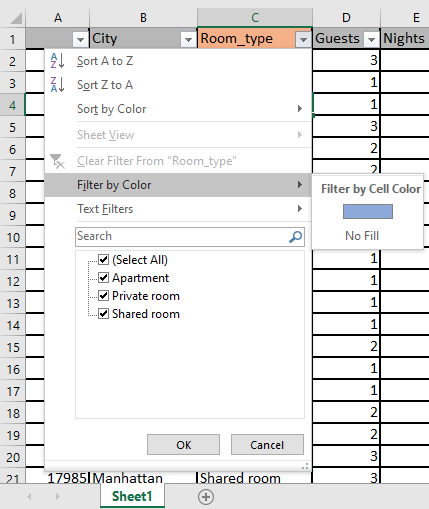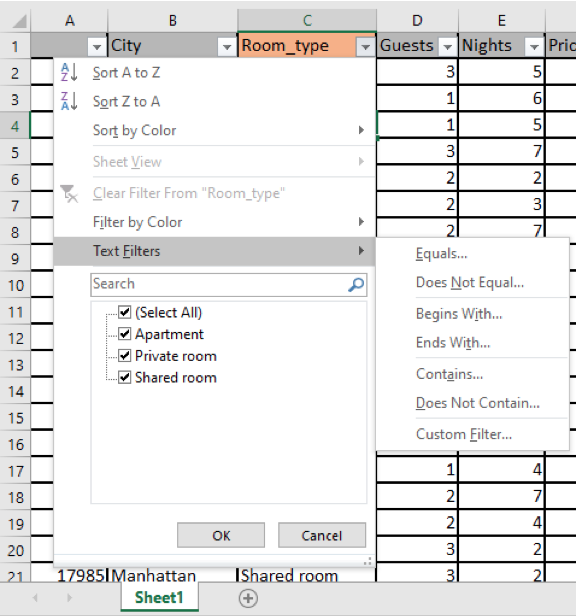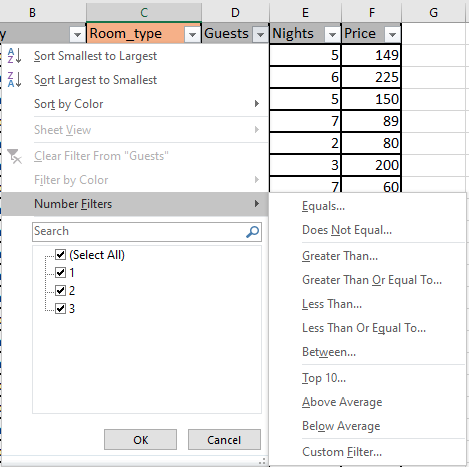# How to Filter by selected cells in ExcelIn this article, we will learn How to Filter by selected cells in Excel.

Scenario:

In Excel, Apply  Filtering is a simple, however, amazing & powerful way to analyze data. Advanced filters are quite easy to use. Here's how you can use Excel's advanced filtering capabilities.

In this article we have created tutorials of advanced filters, in which you can learn how to use formulas and functions for filters and how to use advanced filters. Below you can find different ways of filter.

Filter by in Excel

filter the data as per the selected cell on Excel workbook. Find different filtering options as mentioned below.

Select any table header cell and Access Filter using Home > Sort & Filter > Filter or just use Ctrl + Shift + Enter from keyboard.

You can filter by:

• Cell's value
• Cell's color
• Cell’s font color
• Cell's icon

Example :

All of these might be confusing to understand. Let's understand how to use the function using an example. Here we have a table and we need to see what all types filter an excel user can access.

First of all select any table header and Use Ctrl + Shift + Enter to apply filters. This will enable a drop down option on all column headers.

One of the important features is to filter values by background cell color. This excel option stores all the different color values. Just click the arrow key, this will open the filter table for the selected cell.As you can see here we can filter all blue colored records just by two clicks. Now we learn how to filter by text or numbers value.

Filter by text or number

Excel records text and numbers data separately. In a text column, excel allows only text filters as shown belowHere as you can see there are only text filter values that are allowed to filter. But notice as we switch to the Numbers column it changes to numbers filters as shown below.As you can see the number filter now clearly. You can also access sort values using Colour in Excel.

Here are all the observational notes using the formula in Excel
Notes :

1. You can also access filters using the Filter function (only for new versions) or VBA.
2. Sort & Filter go side by side in Excel. Learn how to use advanced filters in Excel here.

Hope this article about How to Filter by selected cell in Excel is explanatory. Find more articles on calculating values and related Excel formulas here. If you liked our blogs, share it with your friends on Facebook. And also you can follow us on Twitter and Facebook. We would love to hear from you, do let us know how we can improve, complement or innovate our work and make it better for you. Write to us at info@exceltip.com.

Related Articles :

How to Filter the Data in Excel using VBA : Filtering data using VBA is easy. These simple lines of codes filter the data on the given criteria.

How to use the SUBTOTAL function in Excel : Returns the SUM, COUNT, AVERAGE, STDEV or PRODUCT on applied filtered data in Excel.

How to use the Filter Function in Excel 365 (new version) : The FILTER function returns the filtered table by the given column number and condition. The function also works with data in a horizontal manner.

How to use the SORT Function in Excel 365 (new version) : The SORT function  returns the sorted array by the given column number in the array. It also works on horizontal data.

Sort Numeric Values with Excel RANK Function : To sort the numeric values we can use the Rank function. The formula is

Excel Formula to Sort Text : To sort text values using formula in excel we simply use the COUNTIF function. Here is the formula.

How to Delete only Filtered Rows without the Hidden Rows in Excel : Many of you are asking how to delete the selected rows without disturbing the other rows. We will use the Find & Select option in Excel.

Popular Articles :